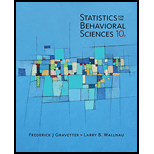# A sample consists of the following n = 7 scores: 5, 0, 4, 5, 1, 2, and 4. a. Compute the mean and standard deviation for the sample. b. Find the z -score for each score in the sample. c. Transform the original sample into a new sample with a mean of M = 50 and s = 10 .### Statistics for The Behavioral Scie...

10th Edition
Frederick J Gravetter + 1 other
Publisher: Cengage Learning
ISBN: 9781305504912### Statistics for The Behavioral Scie...

10th Edition
Frederick J Gravetter + 1 other
Publisher: Cengage Learning
ISBN: 9781305504912

#### Solutions

Chapter
Section
Chapter 5, Problem 26P
Textbook Problem

## Expert Solution

### Want to see the full answer?

Check out a sample textbook solution.See solution

### Want to see this answer and more?

Experts are waiting 24/7 to provide step-by-step solutions in as fast as 30 minutes!*

See Solution

*Response times vary by subject and question complexity. Median response time is 34 minutes and may be longer for new subjects.

Find more solutions based on key concepts
Show solutions
Differentiate the function. y=x3(2+x)

Calculus (MindTap Course List)

Expand each expression in Exercises 122. (xx2)(x+x2)

Finite Mathematics and Applied Calculus (MindTap Course List)

In Exercises 99-106, factor out the greatest common factor from each expression. 104. 2yexy2+2xy3exy2

Applied Calculus for the Managerial, Life, and Social Sciences: A Brief Approach

True or False: xy + 6x2y = 10 x3 is linear.

Study Guide for Stewart's Single Variable Calculus: Early Transcendentals, 8th

Research Methods for the Behavioral Sciences (MindTap Course List)

The better-selling candies are often high in calories. Assume that the following data show the calorie content ...

Modern Business Statistics with Microsoft Office Excel (with XLSTAT Education Edition Printed Access Card) (MindTap Course List)

In Problems 1524, x = 0 is a regular singular point of the given differential equation. Show that the indicial ...

A First Course in Differential Equations with Modeling Applications (MindTap Course List)

TEST YOUR UNDERSTANDING An initial investment of 2000 increases in value by 7 each month. Make a table of value...

Functions and Change: A Modeling Approach to College Algebra (MindTap Course List)